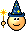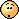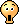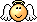Last updated on 3/6/20

## Implement elementary functionsWe're doing great so far. We're learning and practicing a lot by making our program more and more sophisticated - which is wonderful!

Along with our progress, we can observe that our code has grown, which makes it hard to navigate and manage.

A solution for this is using functions.

A function is a named block of code.

We can isolate a number of lines of code and attach a name to it. Then we can call it (refer to it) using that name!

An obvious benefit  of using functions is the ability to organize code for better management.

What block of code should be a function?

My personal rule is - if it's 2 or more lines of code that needs to be repeated at least 2 times - it's destined to be a function!

In addition, if the code becomes too lengthy and hard to navigate - it’s best to put it into a function even if it only needs to be executed once.

Furthermore, if you have a function that completes more than one task: split it up into smaller functions, then make another global function that calls those lesser functions.

#### Function syntax

To declare a function we simply use a keyword  `func`   before its name, followed by parentheses, then curly brackets that surround the block of code that forms our function. Here's an example of a function for greeting a person:

``````func welcome() {
print("Welcome to my awesome application!")
}
``````

To call a function we use its name followed by a parentheses:

``````welcome()
``````

Observe the output in the console:

`Welcome to my awesome application!`

In addition to improving the organization of the code, we can call something multiple times using just one line of code!

Also, if we need to change our welcome message, we only need to do it once by rewriting it in the function itself. Afterwards, if we call it 100 times, the new greeting will appear 100 times!

Isn't it like magic?

It is like magic; that's what software engineering is all about!

Speaking of profit calculation, we can make a function that would calculate the profit for coffee. For example:

``````// declare a function
func coffeeProfit() {
let cost = 0.50 + 1.00
let price = 2.70
let profit = price - cost
print("Profit for one portion of coffee is \$\(profit)")
}

// call previously declared function
coffeeProfit() // Profit for one portion of coffee is \$1.2
``````

Great, we can now create one for each type of item and call an applicable function in each case without having to retype the numbers directly!

Except... the profit variable, which is holding the result of the calculation, is now hidden within our new functionand it's not accessible outside the scope of that function.

And what is scope, now?

Each variable is only available (and accessible) in the context within which it has been declared

To determine the context we can refer to the closest curly brackets that surround the declaration.

Let's experiment:

``````// declare a variable in global scope
var root = "I'm available to all lines of code within my context"

if 2 == 4 / 2 {
// declare a variable within a scope of "if" statement
let spy = "I'm a spy"
print(root) // Ok
print(spy) // Ok
}

// attempt to access a variable declared within "if" statement
print(spy) // Error

let anotherSpy = "I'm another spy"
print(root) // Ok
print(anotherSpy) // Ok

// attempt to access a variable declared within an external "if" statement
print(spy) // Error
}

// attempt to access a variable declared within a function
print(anotherSpy) // Error

print(root) // Ok
``````

As you can observe in Playground, we are not even permitted to use variables out of scope, specifically:  `spy`   and  `anotherSpy` .

Which is exactly what happened to our  `profit`   variable - it's OUT of scope in relation to our original code!

What now?

Now we need to learn another component of functions - a return value

There’s a solution for any problem. Functions may have what’s called a "return value". After executing the block of code, it generates a result and returns it to the line of code where we call that function.

How do we know if a function returns something or not? We can know by its declaration.

When we require a result from a function, we must declare the type of a value the function is expected to return. To do so, we place that type right before the curly brackets that surround the body of our function. Then, in order to provide that expected value we the  `return`  keyword at the end of the code in curly brackets in order to indicate the returning from the function with a value:

``````func calculateSomething() -> Double {
var result = 0.0
// Calculations
return result
}
``````

And then, we simply call that function in order to get the result of its execution:

``````// assign to another variable
var globalResult = calculateSomething()

// icorporate in an output
print("The result of my calculation is \(calculateSomething())")
``````

What value type can a function return?

A function can return ANY value type! This includes all the ones we already know plus some more!

We established that a variable cannot change its type after declaration, remember? However, it may appear to the code as a different type.

Let's see when it can be useful or even necessary. Try this in Playground:

``````let a = 1 + 1.3
print(a) // 2.3
``````

This works fine, Playground is happy, we've got 2.0 printed in console - everything worked as expected.

Now let's modify our code to use variables instead of numbers:

``````let x = 1
let y = 1.3
var a = x + y // Error
print(a)
``````

This didn't go so well...we got an error: "Binary operator '+' cannot be applied to operands of type 'Int' and 'Double'". Rightly so, we already know that we cannot do this in Swift.

What now?

Here's where 'appearance' of a type comes handy. We can make our integer variable appear as Double  instead of Int. We'll use the  `Double()`   function to do that:

``````let x = 1
let y = 1.3
var a = Double(x) + y
print(a) // 2.3
``````

Ah, all good now!

Good to know!

How's this related to functions?

The return value of a function is treated like a variable (it just doesn't have a name until we assign its result to a variable). For example:

``````func generateInt() -> Int {
return 1
}

let y = 1.3
var a = generateInt() + y // Error
print(a)
``````

Like in our attempt with variables, using functions doesn't work in this circumstance. We have to alter the appearance of our integer result:

``````func generateInt() -> Int {
return 1
}

let y = 1.3
var a = Double(generateInt()) + y
print(a) // 2.3
``````

This works perfectly!

Why does this matter?

Remember we've got plenty of code in our SmartBean project that uses direct numbers at the moment!

Finally we can make our "profit calculation" function available in our original code by introducing a return value. To practice, modify our original  `coffeeProfit()`   function to return the result and present the result outside the function:

``````// No cheating!

func coffeeProfit() -> Double {
let cost = 0.50 + 1.00
let price = 2.70
let profit = price - cost
return profit
}

print("Profit for one portion of coffee is \$\(coffeeProfit())")
``````

Well done as always!

Things are getting more and more challenging, great to see you sticking around and learning nontrivial stuff!

Not so fast! A bit more work to do. We will save our final improvements for the next chapter!

• A function is a named block of code.

• Recommended rules to separate some code into a function:

• if it's 2 or more lines of code that needs to be repeated at least 2 times

• if the code becomes too lengthy and hard to navigate even if it only needs to be executed once

• if an existing function completes more than one task it should be split further into smaller functions.

• A function is declared using the  `func`  keyword:

``````func functionName () {
// Implementation
}
``````
• A function can return a value. To create a function with a return value, it must be declared by specifying the type of the expected return value:

``````func functionName () -> Type {
var returnValue
// Implementation
return returnValue
}
``````
• A function can return a value of any type.

• A variable exists only in the context within which it has been declared. This context can be identified by the closest set of curly brackets   `{}`  .